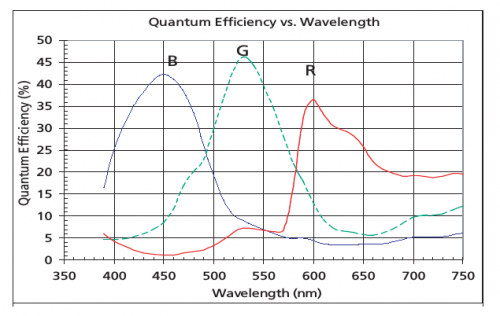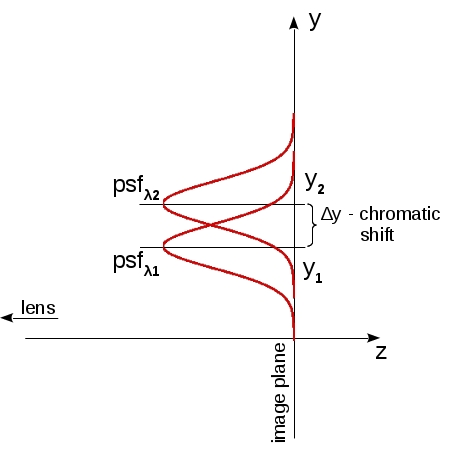July 1, 2014

by Oleg Dzhimiev

Description

The Error Function calculates the 4th root of the average of the 4th power spot sizes over several angles of the field of view.Fig.1 Pixel’s quantum efficiencyFig.2 Example of pixel’s sensitivity range

The function takes into account:

• Pixels’ sensitivity to a band rather than a single wavelength (Fig.1). It negatively affects the sagittal component of the Point Spread Function (PSF).
• One of the goals is the uniform angular resolution and applies the corresponding coefficients to the sagittal component. The angular resolution increases with the field angle increase and degrades with negative distortion amount increase with the field angle increase

Formulas

• If PSF shape is approximated with a Gauss function (Fig.2) (in OSLO actual PSF shapes’s data can be extracted but anyways) then the sagittal PSF for a range of wavelengths will be a Gauss function as well with its Full Width Half Maximum (FWHM) calculated using (5) (Fig.3). FWHM is the spot size.
• With a known frequency for the Modulation Transfer Function (MTF) at the value of 1/2 level FWHM for a single wavelength is calculated with (1)-(4) (Fig.3)
• The final Error Function is shown in (6) (Fig.4). Its value is set as a user-defined operand for minimization (note: the value does not tend to zero).
• The 4th power is used to be able to improve the worst parameters in the first place

Data

• Distortion has not been added yet to the script that sets optimization operands
• Half of the FoV is manually picked at the moment and is 38°
• Field angles are picked to split the circular area of the image plane into the rings (circle in the center) of equal area
• N=6
i αi, rad cos(αi)
1 0.0000 1.0000
2 0.2513 0.9686
3 0.3554 0.9375
4 0.4353 0.9067
5 0.5027 0.8763
6(N) 0.5620 0.8462
Pixel's filter color λpeak,nm range,nm
green 530 510-560
red 600 585-655
blue 450 420-480